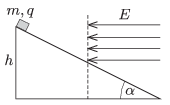Mathematical and Physical Journal
for High Schools
Issued by the MATFUND Foundation
 Already signed up? New to KöMaL?

#Problem P. 4679. (November 2014)

P. 4679. A small object of mass $\displaystyle m$ and of charge $\displaystyle q$ slides down frictionlessly along a slope of angle of elevation $\displaystyle \alpha$ from a height of $\displaystyle h$. From the midpoint of the slope, it enters into a region of uniform horizontal electric field, and it decelerates and stops at the bottom of the slope. Determine the magnitude of the electric field strength $\displaystyle E$.(4 pont)

Deadline expired on December 10, 2014.

### Statistics:

 107 students sent a solution. 4 points: 87 students. 3 points: 17 students. 2 points: 2 students. 1 point: 1 student.

Problems in Physics of KöMaL, November 2014